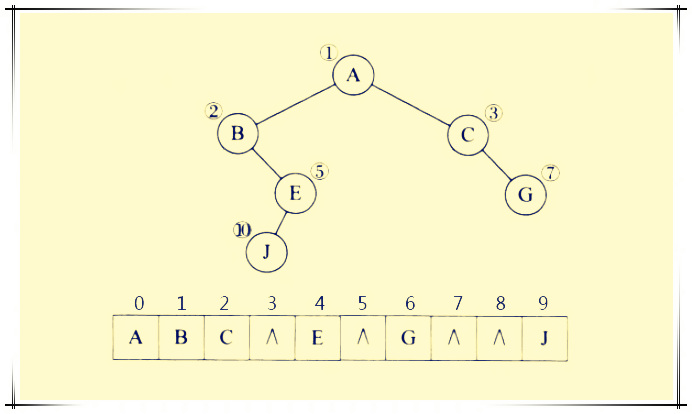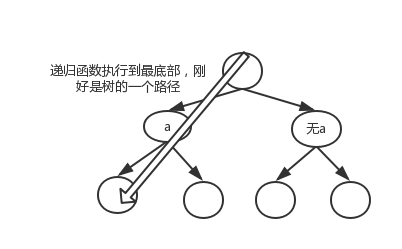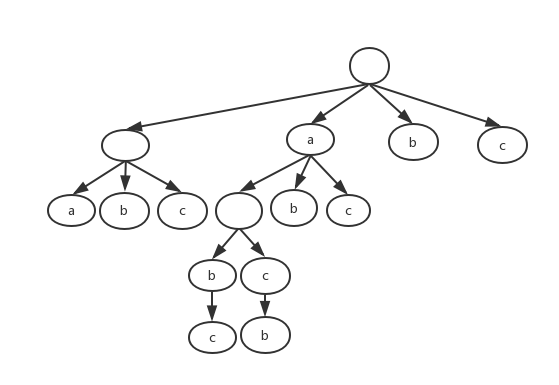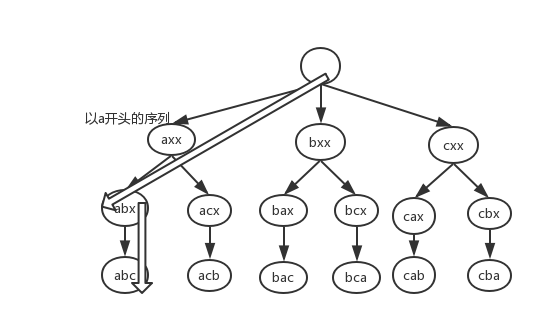2017年10月11日

## 简介

1. 利用字符‘a’、‘b’ 、 ‘c’ 、‘d’ 、‘e’ 、‘f’、‘g’生成并输出所有可能的字符串（但字符不可重复使用，输出顺序无要求），比如： “a”、“b”、“c”、“d”、“e”、“f”、“g”、“ab”、“ba”、“ac”、“ca”

2. 集合A的幂集是由集合A的所有子集所组成的的集合，如：`A=1，2，3`，则A的幂集`P（A）={1，2，3}，{1，2}，{1，3}，{1}，{2，3}，{2}，{3}，{ }`，求一个集合的幂集就是求一个集合的所有的子集。来自回溯法求幂集

## 重新理解递归

1. 看到一个递归实现, 我们总是难免陷入不停的回溯验证之中（把变量的变化依次写出来）, 因为回溯就像反过来思考迭代, 这是我们习惯的思维方式, 但是其实无益于理解。数学归纳法才是理解的递归的方式，函数式编程也有这么点意思。

## 回溯法### 求子集

``````for(i=0;i<len;i++){
for(j=i;j<len;j++){
print(str,i,j);
}
}
``````

``````public class B {
private String str = "abc";
private int[] x = new int;
private void print(int[] x) {
for (int i = 0; i < x.length; i++) {
if (x[i] == 1) {
System.out.print(str.charAt(i));
}
}
System.out.println();
}
public void backtrack(int i) {
/*
x[i] 本质也是个位图，等x[i]从0到len赋值完毕，即可根据位图打印字符串
*/
if (i >= str.length()) {
// 到达叶子节点
print(x);
} else {
for (int isChoose = 0; isChoose <= 1; isChoose++) {
x[i] = isChoose;
backtrack(i + 1);
}
/*相当于
x[i] = 0;
backtrack(i + 1);
x[i] = 1;
backtrack(i + 1);
这就有了二叉树分叉的效果
*/
}
}
public static void main(String[] args) {
new B().backtrack(0);
}
}
``````

``````backtrack{
if (i >= str.length()) {
print(x);
}else{
x[i] = 0;
backtrack(i + 1);
x[i] = 1;
backtrack(i + 1);
}
}
````````````x[i] = 0;
backtrack(i + 1);
x[i] = 1;
backtrack(i + 1);
x[i] = 2;
backtrack(i + 1);
``````

``````private String str = "abc";
private char[] xc = new char;
public void backtrack(int i) {
System.out.println(new String(xc));
if (i >= str.length()) {
return;
} else {
xc[i] = str.charAt(i);
backtrack(i + 1);
xc[i] = 0;
backtrack(i + 1);
}
}
``````

### 全排列``````private String str = "abc";
private int[] x = new int;
private char[] xc = new char;

public void backtrack(int i) {
if (i >= str.length()) {
System.out.println(new String(xc));
} else {
for (int t = 0; t < str.length(); t++) {
// 如果str.charAt(t)还未被选入到xc中
if (x[t] == 0) {
x[t] = 1;
xc[i] = str.charAt(t);
backtrack(i + 1);
x[t] = 0;
xc[i] = 0;
}
}
}
}
````````````private String str = "abc";
public void backtrack(String str, int i) {
if (i >= str.length()) {
System.out.println(str);
} else {
// 每个字符都有当第一个字符的机会，所以n个字符开n个分叉，然后分叉逐渐减少
for (int t = i; t < str.length(); t++) {
str = swap(str, t, i);
backtrack(str,i + 1);
str = swap(str, i, t);
}
}
}
``````

``````// 将index 字符作为首字符
private String firstChar(String str, int index) {
StringBuilder sb = new StringBuilder(str);
sb.deleteCharAt(index);
sb.insert(0, str.charAt(index));
return sb.toString();
}
// 将首字符放在index位置，作为firstChar的反操作
private String _firstChar(String str, int index) {
StringBuilder sb = new StringBuilder(str);
sb.deleteCharAt(0);
sb.insert(index, str.charAt(0));
return sb.toString();
}
public void backtrack(String str, int i) {
if (i >= str.length()) {
System.out.println(str);
} else {
for (int t = i; t < str.length(); t++) {
str = firstChar(str, t);
backtrack(str, i + 1);
str = _firstChar(str, t);
}
}
}
``````

### 子集 + 全排列

``````public void backtrack(int i) {
// 全排列过程中，此时xc还未构造完毕，相当于输出了子集
System.out.println(new String(xc));
if (i >= str.length()) {
return;
} else {
for (int t = 0; t < str.length(); t++) {
if (x[t] == 0) {
x[t] = 1;
xc[i] = str.charAt(t);
backtrack(i + 1);
x[t] = 0;
xc[i] = 0;
}
}
}
}
``````